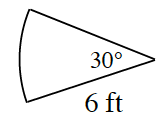### Home > CCG > Chapter 9 > Lesson 9.1.2 > Problem9-27

9-27.

Multiple Choice: Find the perimeter of the sector at right.1. $12π \; \text{ft}$

1. $3π \; \text{ft}$

1. $6 + 3π \; \text{ft}$

1. $12 + π \; \text{ft}$

1. None of these

What fraction of a full circle is this sector?
Find the circumference of the full circle and multiply by this fraction.

$\text{C}=2\pi\text{r}=2\pi\left ( \frac{1}{12} \right )=\pi\text{ units}$

$\text{Perimeter}=\pi+6+6$